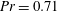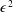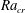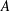Hostname: page-component-546b4f848f-bvkm5 Total loading time: 0 Render date: 2023-06-04T03:50:59.668Z Has data issue: false Feature Flags: { "useRatesEcommerce": true } hasContentIssue false

# Linear and weakly nonlinear analysis of Rayleigh–Bénard convection of perfect gas with non-Oberbeck–Boussinesq effects

Published online by Cambridge University Press:  20 April 2018

*

## Abstract

The influences of non-Oberbeck–Boussinesq (NOB) effects on flow instabilities and bifurcation characteristics of Rayleigh–Bénard convection are examined. The working fluid is air with reference Prandtl number$Pr=0.71$ and contained in two-dimensional rigid cavities of finite aspect ratios. The fluid flow is governed by the low-Mach-number equations, accounting for the NOB effects due to large temperature difference involving flow compressibility and variations of fluid viscosity and thermal conductivity with temperature. The intensity of NOB effects is measured by the dimensionless temperature differential$\unicode[STIX]{x1D716}$. Linear stability analysis of the thermal conduction state is performed. An$\unicode[STIX]{x1D716}^{2}$ scaling of the leading-order corrections of critical Rayleigh number$Ra_{cr}$ and disturbance growth rate$\unicode[STIX]{x1D70E}$ due to NOB effects is identified, which is a consequence of an intrinsic symmetry of the system. The influences of weak NOB effects on flow instabilities are further studied by perturbation expansion of linear stability equations with regard to$\unicode[STIX]{x1D716}$, and then the influence of aspect ratio$A$ is investigated in detail. NOB effects are found to enhance (weaken) flow stability in large (narrow) cavities. Detailed contributions of compressibility, viscosity and buoyancy actions on disturbance kinetic energy growth are identified quantitatively by energy analysis. Besides, a weakly nonlinear theory is developed based on centre-manifold reduction to investigate the NOB influences on bifurcation characteristics near convection onset, and amplitude equations are constructed for both codimension-one and -two cases. Rich bifurcation regimes are observed based on amplitude equations and also confirmed by direct numerical simulation. Weakly nonlinear analysis is useful for organizing and understanding these simulation results.

## JFM classification

Type
JFM Papers
Information
Journal of Fluid Mechanics , 25 June 2018 , pp. 141 - 169

## Access options

Get access to the full version of this content by using one of the access options below. (Log in options will check for institutional or personal access. Content may require purchase if you do not have access.)

## References

Ahlers, G. 1980 Effect of departures from the Oberbeck–Boussinesq approximation on the heat transport of horizontal convecting fluid layers. J. Fluid Mech. 98, 137148.CrossRefGoogle Scholar
Ahlers, G., Brown, E., Araujo, F. F., Funfschilling, D., Grossmann, S. & Lohse, D. 2006 Non-Oberbeck–Boussinesq effects in strongly turbulent Rayleigh–Bénard convection. J. Fluid Mech. 569, 409445.CrossRefGoogle Scholar
Ahlers, G., Dressel, B., Oh, J. & Pesch, W. 2010 Strong non-Boussinesq effects near the onset of convection in a fluid near its critical point. J. Fluid Mech. 642, 1548.CrossRefGoogle Scholar
Ahlers, G., Grossmann, S. & Lohse, D. 2009 Heat transfer and large scale dynamics in turbulent Rayleigh–Bénard convection. Rev. Mod. Phys. 81, 503537.CrossRefGoogle Scholar
Bodenschatz, E., de Bruyn, J. R., Ahlers, G. & Cannell, D. S. 1991 Transitions between patterns in thermal convection. Phys. Rev. Lett. 67, 30783081.CrossRefGoogle ScholarPubMed
Bodenschatz, E., Pesch, W. & Ahlers, G. 2000 Recent developments in Rayleigh–Bénard convection. Annu. Rev. Fluid Mech. 32, 709778.CrossRefGoogle Scholar
Boussinesq, J. 1903 Théorie Analytique de la Chaleur, vol. 2. Gauthier-Villars.Google Scholar
Busse, F. H. 1967 The stability of finite amplitude cellular convection and its relation to an extremum principle. J. Fluid Mech. 30, 625649.CrossRefGoogle Scholar
Carini, M., Auteri, F. & Giannetti, F. 2015 Centre-manifold reduction of bifurcating flows. J. Fluid Mech. 767, 109145.CrossRefGoogle Scholar
Chandrasekhar, S. 1961 Hydrodynamic and Hydromagnetic Stability. Clarendon Press.Google Scholar
Chenoweth, D. R. & Paolucci, S. 1985 Gas flow in vertical slots with large horizontal temperature differences. Phys. Fluids 28, 23652374.CrossRefGoogle Scholar
Chillà, F. & Schumacher, J. 2012 New perspectives in turbulent Rayleigh–Bénard convection. Eur. Phys. J. E 35, 125.Google ScholarPubMed
Ciliberto, S., Coullet, P., Lega, J., Pampaloni, E. & Perez-Garcia, C. 1990 Defects in roll-hexagon competition. Phys. Rev. Lett. 65, 23702373.CrossRefGoogle ScholarPubMed
Ciliberto, S., Pampaloni, E. & Perez-Garcia, C. 1988 Competition between different symmetries in convective patterns. Phys. Rev. Lett. 61, 11981201.CrossRefGoogle ScholarPubMed
Coullet, P. H. & Spiegel, E. A. 1983 Amplitude equations for systems with competing instabilities. SIAM J. Appl. Maths 43, 776821.CrossRefGoogle Scholar
Crawford, J. D. & Knobloch, E. 1991 Symmetry and symmetry-breaking bifurcations in fluid dynamics. Annu. Rev. Fluid Mech. 23, 341387.CrossRefGoogle Scholar
Cross, M. C. & Hohenberg, P. C. 1993 Pattern formation outside of equilibrium. Rev. Mod. Phys. 65, 8511112.CrossRefGoogle Scholar
Fröhlich, J., Laure, P. & Peyret, R. 1992 Large departures from Boussinesq approximation in the Rayleigh–Bénard problem. Phys. Fluids 4, 13551372.CrossRefGoogle Scholar
Gao, P. & Lu, X. Y. 2006 Instability of channel flow with oscillatory wall suction/blowing. Phys. Fluids 18, 034102.CrossRefGoogle Scholar
Golubitsky, M., Stewart, I. N. & Schaeffer, D. G. 1988 Singularities and Groups in Bifurcation Theory, vol. 2. Springer.CrossRefGoogle Scholar
Gray, D. D. & Giorgini, A. 1976 The validity of the Boussinesq approximation for liquids and gases. Intl J. Heat Mass Transfer 19, 545551.CrossRefGoogle Scholar
Horn, S. & Shishkina, O. 2014 Rotating non-Oberbeck–Boussinesq Rayleigh–Bénard convection in water. Phys. Fluids 26 (5), 055111.CrossRefGoogle Scholar
Horn, S., Shishkina, O. & Wagner, C. 2013 On non-Oberbeck–Boussinesq effects in three-dimensional Rayleigh–Bénard convection in glycerol. J. Fluid Mech. 724, 175202.CrossRefGoogle Scholar
Hoyle, R. 2006 Pattern Formation: An Introduction to Methods. Cambridge University Press.CrossRefGoogle Scholar
Ma, D. J., Henry, D. & Hadid, H. B. 2005 Three-dimensional numerical study of natural convection in vertical cylinders partially heated from the side. Phys. Fluids 17, 124101.CrossRefGoogle Scholar
Metzener, P. 1986 The effect of rigid sidewalls on nonlinear two-dimensional Bénard convection. Phys. Fluids 29, 13731377.CrossRefGoogle Scholar
Mizushima, J. 1995 Onset of the thermal convection in a finite two-dimensional box. J. Phys. Soc. Japan 64, 24202432.CrossRefGoogle Scholar
Normand, C., Pomeau, Y. & Velarde, M. G. 1977 Convective instability: a physicist’s approach. Rev. Mod. Phys. 49, 581624.CrossRefGoogle Scholar
Oberbeck, A. 1879 Über die Wärmeleitung der Flüssigkeiten bei Berücksichtigung der Strömungen infolge von Temperaturdifferenzen. Ann. Phys. 243, 271292.CrossRefGoogle Scholar
Pampaloni, E., Perez-Garcia, C., Albavetti, L. & Ciliberto, S. 1992 Transition from hexagons to rolls in convection in fluids under non-Boussinesq conditions. J. Fluid Mech. 234, 393416.CrossRefGoogle Scholar
Paolucci, S.1982 Filtering of sound from the Navier–Stokes equations. Tech. Rep. Sandia National Laboratories, Livermore.Google Scholar
Paolucci, S. & Chenoweth, D. R. 1987 Departures from the Boussinesq approximation in laminar Bénard convection. Phys. Fluids 30, 15611564.CrossRefGoogle Scholar
Robinson, F. & Chan, K. 2004 Non-Boussinesq simulations of Rayleigh–Bénard convection in a perfect gas. Phys. Fluids 16, 13211333.CrossRefGoogle Scholar
Sugiyama, K., Calzavarini, E., Grossmann, S. & Lohse, D. 2007 Non-Oberbeck–Boussinesq effects in two-dimensional Rayleigh–Bénard convection in glycerol. Europhys. Lett. 80 (3), 34002.CrossRefGoogle Scholar
Suslov, S. A. & Paolucci, S. 1995 Stability of mixed-convection flow in a tall vertical channel under non-Boussinesq conditions. J. Fluid Mech. 302, 91115.CrossRefGoogle Scholar
Suslov, S. A. & Paolucci, S. 1997 Nonlinear analysis of convection flow in a tall vertical enclosure under non-Boussinesq conditions. J. Fluid Mech. 344, 141.CrossRefGoogle Scholar
Suslov, S. A. & Paolucci, S. 1999a Nonlinear stability of mixed convection flow under non-Boussinesq conditions. Part 1. Analysis and bifurcations. J. Fluid Mech. 398, 6185.CrossRefGoogle Scholar
Suslov, S. A. & Paolucci, S. 1999b Nonlinear stability of mixed convection flow under non-Boussinesq conditions. Part 2. Mean flow characteristics. J. Fluid Mech. 398, 87108.CrossRefGoogle Scholar
Trefethen, L. N. 2000 Spectral Methods in MATLAB. SIAM.CrossRefGoogle Scholar
Wang, B. F., Ma, D. J., Chen, C. & Sun, D. J. 2012 Linear stability analysis of cylindrical Rayleigh–Bénard convection. J. Fluid Mech. 711, 2739.CrossRefGoogle Scholar
Wang, B. F., Wan, Z. H., Guo, Z. W., Ma, D. J. & Sun, D. J. 2014 Linear instability analysis of convection in a laterally heated cylinder. J. Fluid Mech. 747, 447459.CrossRefGoogle Scholar
Wang, S. X. 2008 A novel method for analyzing the global stability of inviscid columnar swirling flow in a finite pipe. Phys. Fluids 20, 074101.CrossRefGoogle Scholar
Xia, S. N., Wan, Z. H., Liu, S., Wang, Q. & Sun, D. J. 2016 Flow reversals in Rayleigh–Bénard convection with non-Oberbeck–Boussinesq effects. J. Fluid Mech. 798, 628642.Google Scholar